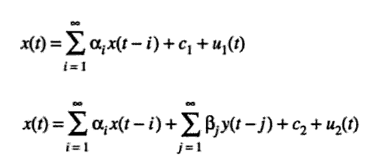# Granger Causality: Definition, Running the Test

Hypothesis Tests > Granger Causality

## What is Granger causality?

Granger causality is a way to investigate causality between two variables in a time series. The method is a probabilistic account of causality; it uses empirical data sets to find patterns of correlation.

Causality is closely related to the idea of cause-and-effect, although it isn’t exactly the same. A variable X is causal to variable Y if X is the cause of Y or Y is the cause of X. However, with Granger causality, you aren’t testing a true cause-and-effect relationship; What you want to know is if a particular variable comes before another in the time series. In other words, if you find Granger causality in your data there isn’t a causal link in the true sense of the word (for example, sales of Easter baskets Granger-cause Easter!). Note: When econometricians say “cause,” what they mean is “Granger-cause,” although a more appropriate word might be “precedence” (Leamer, 1985).

## Bottom Up / Top Down

Granger causality is a “bottom up” procedure, where the assumption is that the data-generating processes in any time series are independent variables; then the data sets are analyzed to see if they are correlated. The opposite is a “top down” method which assumes the processes are not independent; the data sets are then analyzed to see if they are generated independently from each other.

## Running the Test

The null hypothesis for the test is that lagged x-values do not explain the variation in y. In other words, it assumes that x(t) doesn’t Granger-cause y(t). Theoretically, you can run the Granger Test to find out if two variables are related at an instantaneous moment in time. However, that version of the test is seldom used because it’s not very useful, so I have not included the steps here.

## Steps for the F-Test

The procedure can get complex because of the large number of options, including choosing from a set of equations for the f-value calculations. You can skip the vast majority of the intermediate steps by using software. The Granger causality test is part of many popular economics software packages, including E-Views. Any number of lags can be selected with a few clicks.

Make sure your time series is stationary before proceeding. Data should be transformed to eliminate the possibility of autocorrelation. You should also make sure your model doesn’t have any unit roots, as these will skew the test results.

The basic steps for running the test are:

1. State the null hypothesis and alternate hypothesis. For example, y(t) does not Granger-cause x(t).
2. Choose the lags. This mostly depends on how much data you have available. One way to choose lags i and j is to run a model order test (i.e. use a model order selection method). It might be easier just to pick several values and run the Granger test several times to see if the results are the same for different lag levels. The results should not be sensitive to lags.
3. Find the f-value. Two equations can be used to find if βj = 0 for all lags j:

Similarly, these equations test to see if y(t) Granger-causes x(t):4. Calculate the f-statistic using the following equation:5. Reject the null if the F statistic (Step 4) is greater than the f-value (Step 3).

## Alternative Test

If you have a large number of variables and lags, your F-test can lose power. An alternative would be to run a chi-square test, constructed with likelihood ratio or Wald tests. Although both versions give practically the same result, the F-test is much easier to run.

References
Cromwell, J. et. al. (1994) Multivariate Tests for Time Series Models, Issue 100. Sage University.
Granger, C. (1969). Investigating Causal Relations by Econometric Models and Cross-Spectral Methods. Econometrica, Volume 37, Issue 3 (Aug). Available from here.
Hoover, K. (2001) Causality in Macroeconomics. Cambridge University Press.
Leamer, E. (1985) Vector Autoregressions for Causal Inference?, in K. Brunner – A.H. Meltzer (a cura di), Understanding Monetary Regime, Carnegie-Rochester Conference Series on Public Policy, 22, pp. 255-304.

CITE THIS AS:
Stephanie Glen. "Granger Causality: Definition, Running the Test" From StatisticsHowTo.com: Elementary Statistics for the rest of us! https://www.statisticshowto.com/granger-causality/